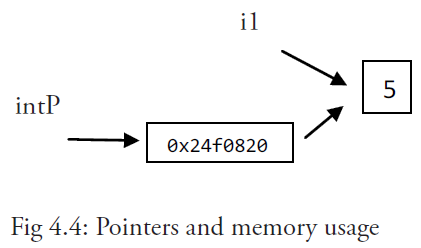# 4.9 指针

Go 语言的取地址符是 `&`，放到一个变量前使用就会返回相应变量的内存地址。

``````var i1 = 5
fmt.Printf("An integer: %d, it's location in memory: %p\n", i1, &i1)
``````

``````var intP *int
``````

（指针的格式化标识符为 `%p`

intP 存储了 i1 的内存地址；它指向了 i1 的位置，它引用了变量 i1。

``````package main
import "fmt"
func main() {
var i1 = 5
fmt.Printf("An integer: %d, its location in memory: %p\n", i1, &i1)
var intP *int
intP = &i1
fmt.Printf("The value at memory location %p is %d\n", intP, *intP)
}
``````

``````An integer: 5, its location in memory: 0x24f0820
The value at memory location 0x24f0820 is 5
````````````package main
import "fmt"
func main() {
s := "good bye"
var p *string = &s
*p = "ciao"
fmt.Printf("Here is the pointer p: %p\n", p) // prints address
fmt.Printf("Here is the string *p: %s\n", *p) // prints string
fmt.Printf("Here is the string s: %s\n", s) // prints same string
}
``````

``````Here is the pointer p: 0x2540820
Here is the string *p: ciao
Here is the string s: ciao
````````````const i = 5
ptr := &i //error: cannot take the address of i
ptr2 := &10 //error: cannot take the address of 10
``````

``````package main
func main() {
var p *int = nil
*p = 0
}
// in Windows: stops only with: <exit code="-1073741819" msg="process crashed"/>
// runtime error: invalid memory address or nil pointer dereference
``````